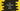# 4 ways to concatenate two arrays in C sharp## How to concatenate two arrays in C#:

In this post, we will learn how to concatenate or merge two arrays in C#. There are different ways we can use to find the array concatenation. Let’s learn these methods with examples:

### Method 1: By appending the array elements to a list:

We have methods to append the array elements to a list. We can also convert a list to an array. So, we can append the elements of both arrays to a list and convert the list to an array to get the final array.

``````namespace Example
{
class Program
{
static void Main(string[] args)
{
int[] FirstArray = { 1, 2, 3, 4, 5 };
int[] SecondArray = { 6, 7, 8, 9 };

var ResultList = new List<int>();

int[] ResultArray = ResultList.ToArray();

Console.WriteLine("First Array: " + String.Join(",", FirstArray));
Console.WriteLine("Second Array: " + String.Join(",", SecondArray));
Console.WriteLine("Final Array: " + String.Join(",", ResultArray));
}
}
}``````

Here,

• FirstArray is the first array and the SecondArray is the second array. Both are arrays of integers.
• One empty list, ResultList is created to hold the final result.
• The AddRange method appends the content of the array to the list. We are using AddRage with both of these arrays to add the contents to the list.
• The ToArray() method converts the list to an array. It returns an array and it is assigned to the ResultArray variable.

If you run this program, it will print the below output:

``````First Array: 1,2,3,4,5
Second Array: 6,7,8,9
Final Array: 1,2,3,4,5,6,7,8,9``````

### Method 2: By using Enumerable.Concat method:

The Enumerable.Concat method can be used to concatenate the content of multiple arrays. This method returns one enumerable, so we have to use ToArray to convert the result back to an array.

``````namespace Example
{
class Program
{
static void Main(string[] args)
{
int[] FirstArray = { 1, 2, 3, 4, 5 };
int[] SecondArray = { 6, 7, 8, 9 };

int[] ResultArray = FirstArray.Concat(SecondArray).ToArray();

Console.WriteLine("First Array: " + String.Join(",", FirstArray));
Console.WriteLine("Second Array: " + String.Join(",", SecondArray));
Console.WriteLine("Final Array: " + String.Join(",", ResultArray));
}
}
}``````

This is similar to the above program. If you run it, it will print the same result.

### Method 3: By using Array.CopyTo() method:

The Array.CopyTo method copies the content of one array to another array starting from a given index. This is defined as like below:

``public void CopyTo (Array arr, long i);``

Here, arr is the destination array to copy the contents. i is the index to start the copy. We have to create one array with a size equal to the total size of both arrays. This array will hold the result. Then, we can use CopyTo to copy the contents of the arrays.

``````namespace Example
{
class Program
{
static void Main(string[] args)
{
int[] FirstArray = { 1, 2, 3, 4, 5 };
int[] SecondArray = { 6, 7, 8, 9 };
int[] ResultArray = new int[FirstArray.Length + SecondArray.Length];

FirstArray.CopyTo(ResultArray, 0);
SecondArray.CopyTo(ResultArray, FirstArray.Length);

Console.WriteLine("First Array: " + String.Join(",", FirstArray));
Console.WriteLine("Second Array: " + String.Join(",", SecondArray));
Console.WriteLine("Final Array: " + String.Join(",", ResultArray));
}
}
}``````
• The ResultArray is the final array to hold the result.
• The CopyTo method is used to copy the contents of the FirstArray and SecondArray to ResultArray.

If you run this program, it will print a similar result.

``````First Array: 1,2,3,4,5
Second Array: 6,7,8,9
Final Array: 1,2,3,4,5,6,7,8,9``````

### Method 4: By using Array.Copy and Array.Resize:

The Array.Copy method copies a range of elements from one array to another. The Array.Resize method is used to change the number of elements of one array to a new size.

We can use Array.Resize to change the size of the first array to the total size of both arrays and Array.Copy can be used to copy the content of the second array to the end of the first array.

``````namespace Example
{
class Program
{
static void Main(string[] args)
{
int[] FirstArray = { 1, 2, 3, 4, 5 };
int[] SecondArray = { 6, 7, 8, 9 };
int TotalSize = FirstArray.Length + SecondArray.Length;
int FirstArrayInitialLength = FirstArray.Length;
int SecondArrayInitialLength = SecondArray.Length;

Console.WriteLine("First Array: " + String.Join(",", FirstArray));
Console.WriteLine("Second Array: " + String.Join(",", SecondArray));

Array.Resize(ref FirstArray, TotalSize);
Array.Copy(SecondArray, 0, FirstArray, FirstArrayInitialLength, SecondArrayInitialLength);

Console.WriteLine("Final Array: " + String.Join(",", FirstArray));
}
}
}``````

Here,

• The TotalSize variable is the total size of the first and the second array.
• FirstArrayInitialLength is the initial length of the first array. Since we will modify the length of the first array to hold the result, its initial length is calculated in the beginning.
• Similarly, SecondArrayInitialLength is the initial length of the second array.
• The Array.Resize method is changing the size of the FirstArray to the final size, i.e. TotalSize.
• The Array.Copy method is copying the content of the SecondArray to the end of the FirstArray.

If you run this program, it will print a similar result.